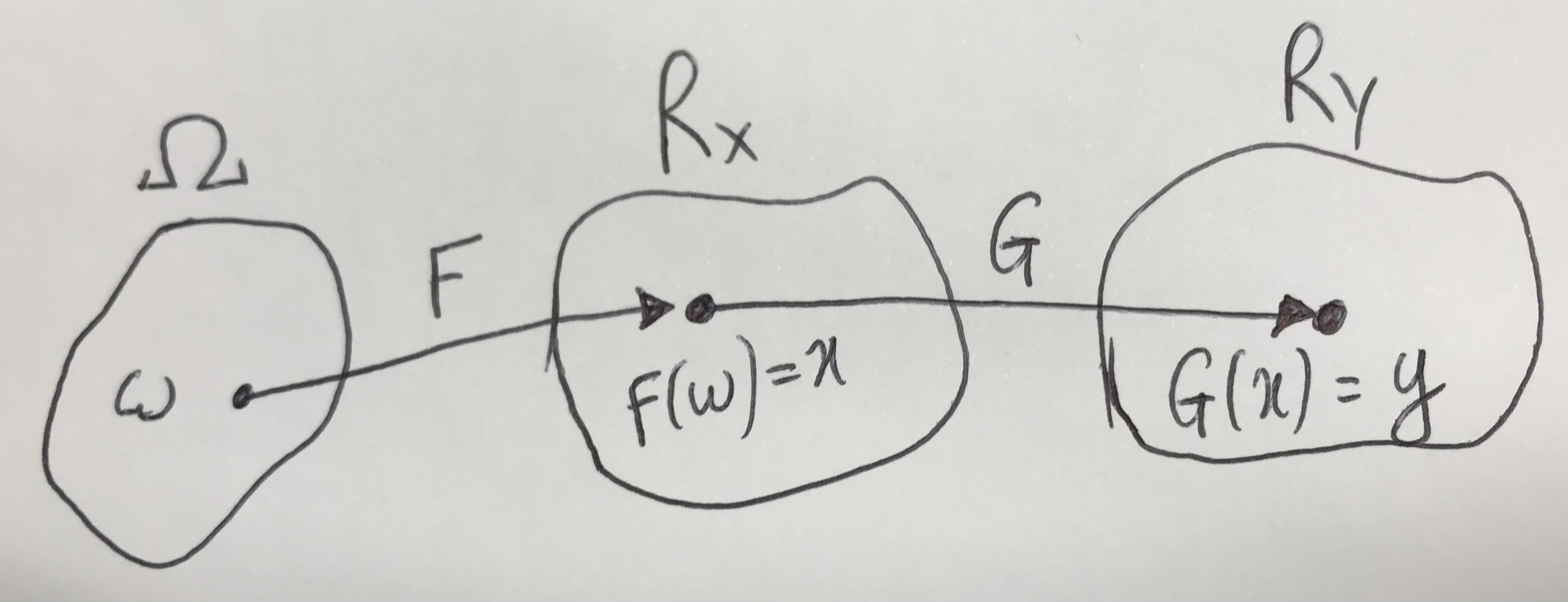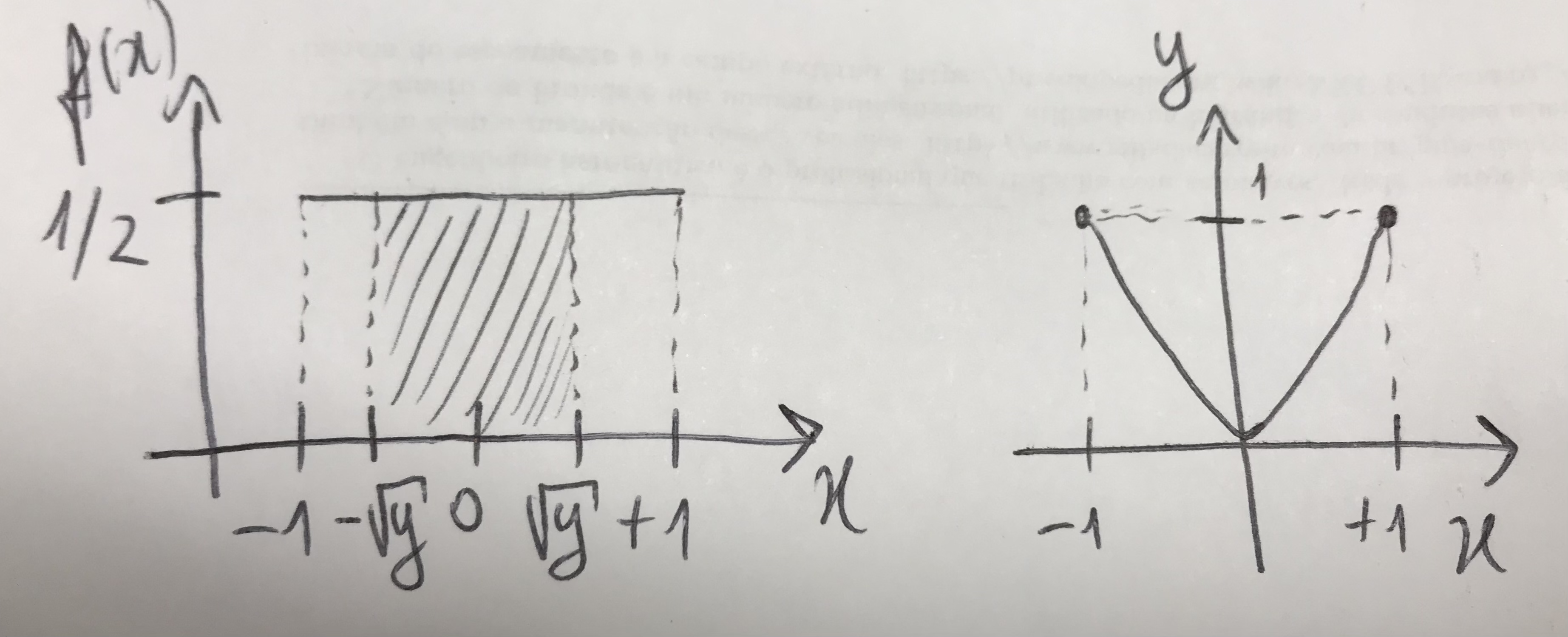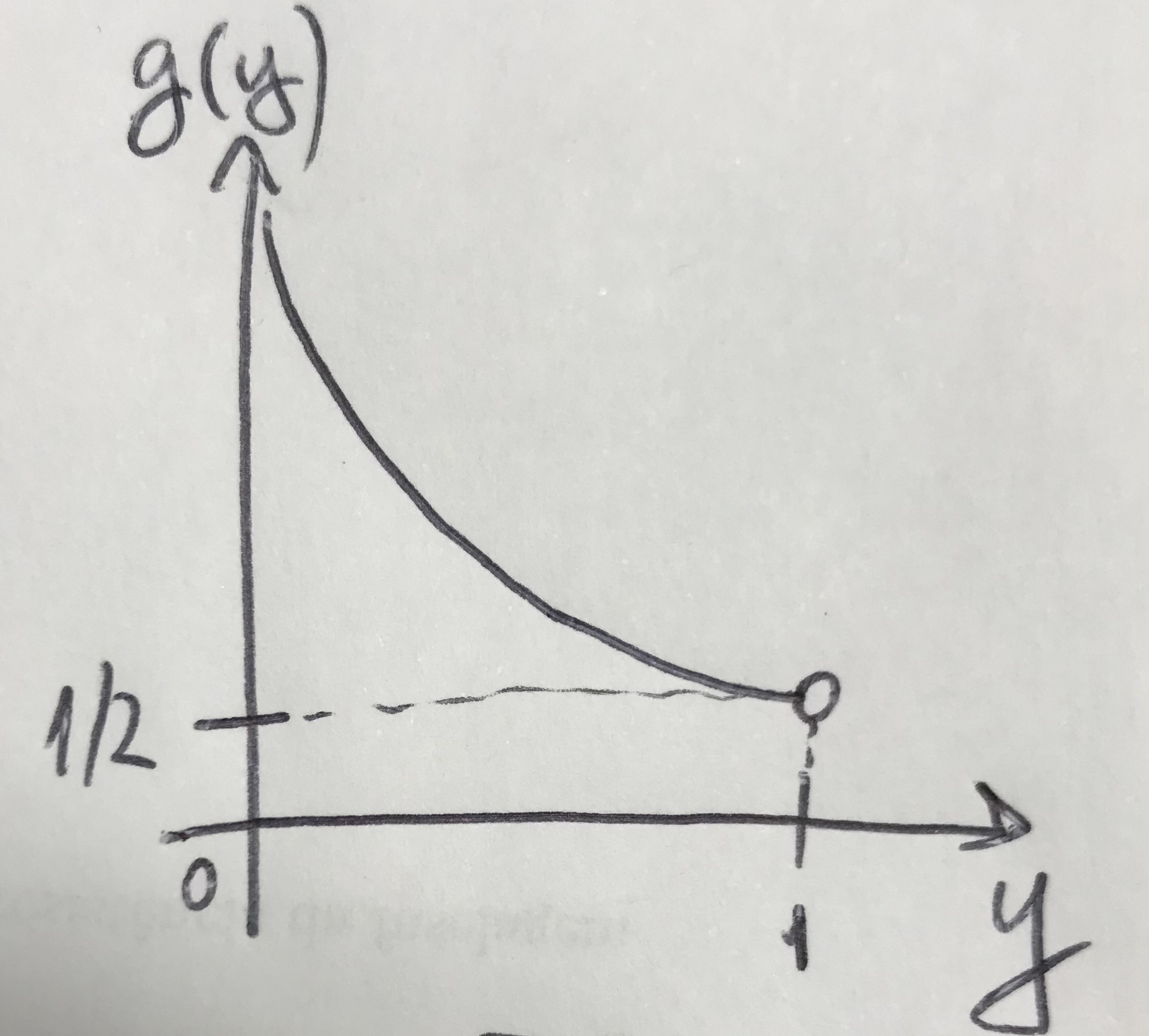## 3.11 Functions of random variables

A function of random variables is a transformation (function) of $$\mathbb{R}^n$$ into $$\mathbb{R}^n$$, denoted $$g:\mathbb{R}^n \rightarrow \mathbb{R}^n$$.Example 3.46 Let the random variable be $$X \sim \mathcal{U}(-1,1)$$ and the function $$Y=X^2$$. It is possible to obtain the pdf $$g(y)$$. Initially consider the graphs of $$f(x)$$ by $$x$$ and $$y$$ by $$x$$. From the graphs it is possible to verify that $$x \in (-1,1)$$, $$f(x)=\frac{1}{2}$$ and $$y \in (0,1)$$. The limits $$-\sqrt{y}$$ and $$\sqrt{y}$$ are obtained during Step 1 below.Step 1

\begin{align*} G(y) &= Pr(Y < y) \\ &= Pr(X^2 < y) \\ &= Pr(-\sqrt{y} < X < \sqrt{y}) \\ &= \int_{-\sqrt{y}}^{\sqrt{y}} \frac{1}{2} dx \\ &= \frac{1}{2} [\sqrt{y} - (-\sqrt{y})] \\ G(y) &= \sqrt{y} \end{align*}

Checking
$$G(0) = \sqrt{0} = 0$$
$$G(1) = \sqrt{1} = 1$$

Step 2

\begin{align*} g(y) &= G'(y) \\ &= \frac{1}{2} y^{\frac{1}{2}-1} \\ &= \frac{1}{2 \sqrt{y}}\\ g(y) &= \frac{\sqrt{y}}{2y} \end{align*}

Checking
$$\int_{0}^{1} \frac{1}{2} y^{-\frac{1}{2}} dy = \frac{1}{2}[2y^{\frac{1}{2}}] \Big|_0^1 = 1$$

Finally, it is possible to graph $$g(y)$$ by $$y$$, verifying $$g(y) \in (1/2,\infty)$$.